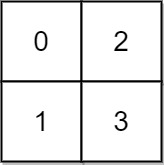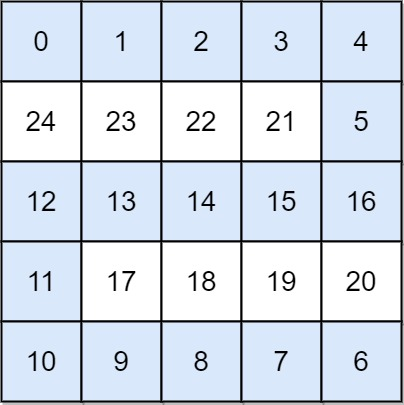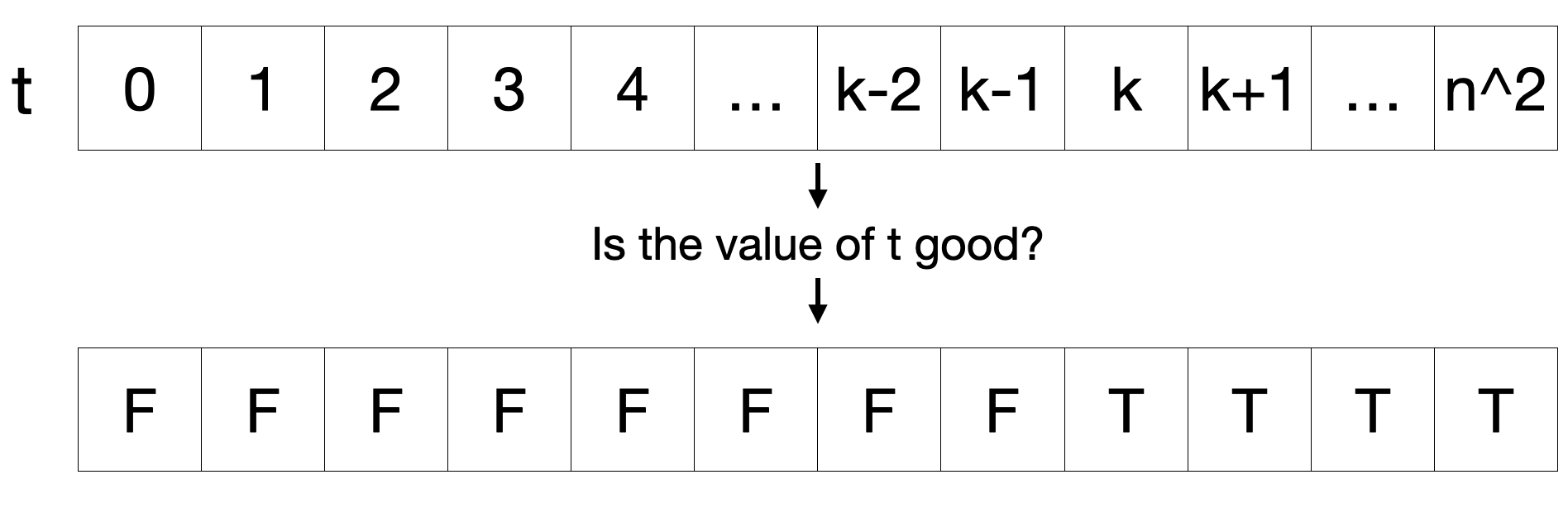# LeetCode 778. Swim in Rising Water Solution

You are given an n x n integer matrix grid where each value grid[i][j] represents the elevation at that point (i, j).

The rain starts to fall. At time t, the depth of the water everywhere is t. You can swim from a square to another 4-directionally adjacent square if and only if the elevation of both squares individually are at most t. You can swim infinite distances in zero time. Of course, you must stay within the boundaries of the grid during your swim.

Return the least time until you can reach the bottom right square (n - 1, n - 1) if you start at the top left square (0, 0).

Example 1:Input: grid = [[0,2],[1,3]]
Output: $3$
Explanation:
At time $0$, you are in grid location (0, 0). You cannot go anywhere else because 4-directionally adjacent neighbors have a higher elevation than t = 0. You cannot reach point (1, 1) until time $3$. When the depth of water is $3$, we can swim anywhere inside the grid.

Example 2:Input: grid = [[0,1,2,3,4],[24,23,22,21,5],[12,13,14,15,16],[11,17,18,19,20],[10,9,8,7,6]]
Output: $16$
Explanation: The final route is shown.
We need to wait until time $16$ so that (0, 0) and (4, 4) are connected.

Constraints:

• n == grid.length
• n == grid[i].length
• $1 \leq n \leq 50$
• $0\leq$ grid[i][j] $< n^2$
• Each value grid[i][j] is unique.

## Brute Force

In simpler terms, this problem is asking us what's the minimum value of $t$ such that you can travel from the top-left square to the bottom-right square by only travelling between adjacent cells that never exceed an elevation of $t$. To solve this problem, we can try all values of $t$ from $0$ to $n^2-1$. For each value of $t$, we can run a BFS/flood fill algorithm to check the connectivity between the top-left and bottom-right squares. It's important that in this algorithm, we only traverse squares with elevations that don't exceed $t$.

Since there are $\mathcal{O}(N^2)$ values of $t$ to try and our BFS/flood fill algorithm runs in $\mathcal{O}(N^2)$, this gives us a time complexity of $\mathcal{O}(N^4)$.

## Full Solution

Let's denote a value $t$ as good if the bottom-right square is reachable from the top-left square only through squares with elevations not exceeding $t$. Let's denote the minimum good value $t$ as $k$. We can observe that all values $t$ such that $t < k$ are never good and that all values $t$ such that $t\geq k$ are all good. What does this mean for us? It means we can binary search it since our binary search condition is satisfied.Each binary search iteration, we'll use the same BFS/flood fill algorithm to check if a specific value of $t$ is good.

### Time Complexity

Note that $\log{N^2} = 2\log{N}$ so $\mathcal{O}(\log{N^2}) = \mathcal{O}(\log{N})$.

We have $\mathcal{O}(\log{N})$ binary search iterations and each iteration uses a BFS/flood fill algorithm which runs in $\mathcal{O}(N^2)$. Thus, our final time complexity is $\mathcal{O}(N^2\log{N})$.

Time Complexity: $\mathcal{O}(N^2\log{N})$

### Space Complexity

Our BFS/flood fill algorithm will take $\mathcal{O}(N^2)$ space so our space complexity is $\mathcal{O}(N^2)$.

Space Complexity: $\mathcal{O}(N^2)$

## C++ Solution

1class Solution {
2    const vector<int> deltaRow = {-1, 0, 1, 0};
3    const vector<int> deltaCol = {0, 1, 0, -1};
4    bool isEndReachable(vector<vector<int>>& grid, int t) {
5        if (grid > t) {  // starting elevation can't exceed t
6            return false;
7        }
8        int n = grid.size();
9        vector<vector<bool>> vis(n, vector<bool>(n));
10        queue<vector<int>> q;
11        q.push({0, 0});  // starting cell
12        vis = true;
13        while (!q.empty()) {
14            vector<int> cur = q.front();
15            q.pop();
16            int curRow = cur;
17            int curCol = cur;
18            for (int i = 0; i < 4; i++) {
19                int newRow = curRow + deltaRow[i];
20                int newCol = curCol + deltaCol[i];
21                if (newRow < 0 || newRow >= n || newCol < 0 || newCol >= n) {  // outside of boundary
22                    continue;
23                }
24                if (vis[newRow][newCol]) {  // visited node before
25                    continue;
26                }
27                if (grid[newRow][newCol] > t) {  // check if cell can be traversed
28                    continue;
29                }
30                vis[newRow][newCol] = true;
31                q.push({newRow, newCol});
32            }
33        }
34        return vis[n - 1][n - 1];
35    }
36
37   public:
38    int swimInWater(vector<vector<int>>& grid) {
39        int n = grid.size();
40        int low = -1;      // all values smaller or equal to low are not good
41        int high = n * n;  // all values greater or equal to high are good
42        int mid = (low + high) / 2;
43        while (low + 1 < high) {
44            if (isEndReachable(grid, mid)) {
45                high = mid;
46            } else {
47                low = mid;
48            }
49            mid = (low + high) / 2;
50        }
51        return high;
52    }
53};

## Java Solution

1class Solution {
2    static int[] deltaRow = {-1, 0, 1, 0};
3    static int[] deltaCol = {0, 1, 0, -1};
4    boolean isEndReachable(int[][] grid, int t) {
5        if (grid > t) { // starting elevation can't exceed t
6            return false;
7        }
8        int n = grid.length;
9        boolean[][] vis = new boolean[n][n];
10        Queue<int[]> q = new LinkedList<int[]>();
11        int[] start = {0, 0}; // starting cell
13        vis = true;
14        while (!q.isEmpty()) {
15            int[] cur = q.poll();
16            int curRow = cur;
17            int curCol = cur;
18            for (int i = 0; i < 4; i++) {
19                int newRow = curRow + deltaRow[i];
20                int newCol = curCol + deltaCol[i];
21                if (newRow < 0 || newRow >= n || newCol < 0 || newCol >= n) { // outside of boundary
22                    continue;
23                }
24                if (vis[newRow][newCol]) { // visited node before
25                    continue;
26                }
27                if (grid[newRow][newCol] > t) { // check if cell can be traversed
28                    continue;
29                }
30                vis[newRow][newCol] = true;
31                int[] destination = {newRow, newCol};
33            }
34        }
35        return vis[n - 1][n - 1];
36    }
37    public int swimInWater(int[][] grid) {
38        int n = grid.length;
39        int low = -1; // all values smaller or equal to low are not good
40        int high = n * n; // all values greater or equal to high are good
41        int mid = (low + high) / 2;
42        while (low + 1 < high) {
43            if (isEndReachable(grid, mid)) {
44                high = mid;
45            } else {
46                low = mid;
47            }
48            mid = (low + high) / 2;
49        }
50        return high;
51    }
52}

## Python Solution

1class Solution:
2    def swimInWater(self, grid: List[List[int]]) -> int:
3        deltaRow = [-1, 0, 1, 0]
4        deltaCol = [0, 1, 0, -1]
5
6        def isEndReachable(grid, t):
7            if grid > t:  # starting elevation can't exceed t
8                return False
9            n = len(grid)
10            vis = [[False] * n for a in range(n)]
11            q = [(0, 0)]  # starting cell
12            vis = True
13            while len(q):
14                (curRow, curCol) = q.pop()
15                for i in range(4):
16                    newRow = curRow + deltaRow[i]
17                    newCol = curCol + deltaCol[i]
18                    if newRow < 0 or newRow >= n or newCol < 0 or newCol >= n:
19                        # outside of boundary
20                        continue
21                    if vis[newRow][newCol]:  # visited node before
22                        continue
23                    if grid[newRow][newCol] > t:  # check if cell can be traversed
24                        continue
25                    vis[newRow][newCol] = True
26                    q.append([newRow, newCol])
27            return vis[n - 1][n - 1]
28
29        n = len(grid)
30        low = -1  # all values smaller or equal to low are not good
31        high = n * n  # all values greater or equal to high are good
32        mid = (low + high) // 2
33        while low + 1 < high:
34            if isEndReachable(grid, mid):
35                high = mid
36            else:
37                low = mid
38            mid = (low + high) // 2
39        return high
40